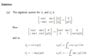# ODE question: Understanding a step in the solution

• Bonnie

## Homework Statement

Hi there, I don't nee help with solving a question, so much as understanding a step in the provided worked solution. It's using variation of parameters to solve the ode y''+ y = g(t). I've attached the steps in the picture file, and the bit after the word 'Now' what are they doing to the first matrix in order to get the one following the arrow? It might be really obvious but I don't quite get what they've done.

Aso, since y1 = eit and y2 = e-it, shouldn't the terms in the very first matrix listed (before the ones I referred to earlier) be cos(t)+isin(t) (eg. for the first entry) etc, instead of just cos(t)?
Many thanks

## The Attempt at a Solution

#### Attachments

•VoP.JPG
11.5 KB · Views: 219
Last edited:
I could not follow the second step=the notation seems unclear, but the first matrix has a very obvious inverse if you are familiar with the equations that do a rotation of axes. Using this it is very easy to get the identity matrix on the left side that is multiplied by the column vector ## (u_1', u_2') ##.

what are they doing to the first matrix in order to get the one following the arrow?
Gaussian elimination

y1 = eit and y2 = e-it
The author of the solution used different expressions for ##y_1## and ##y_2##. Given the matrices in your attachment, you should be able to guess what they are.

•